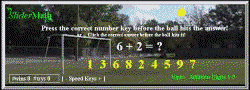< WIDTH="260" ALIGN="left">Teaching & Testing  Help for Math View Page 2:   PolynomialsGraphs+Equations+Trigonometry

Sequenced Math Help Games
SliderMath.com Games. For juniors & seniors. For computers, cell phones & tablets.
Practice and maintain your skills from the convenience of your home.Arithmetic Teaching Fun - Grades 1&2 - Add, Subtract: Addition Themes:  Soccer Addition  Baseball Addition  Basketball Addition  Tennis Addition   Find part of a Sum   Add 3 numbers Subtraction:  (digits 1-9)   (digits 0-20)   Add & Subtract Fun Math Help Games - Grades 3&4 - Multiply, Perimeter, Area:   Given Sums to 21   Place Value   Read Bar Graphs Multiply 2x  3x  4x  5x  6x  7x  7by7   Venn Diagram Sets Round to Tens   Perimeter   Area   Equivalent Fractions Math Help Games - Grades 5&6 - Division, Decimal Fractions, Distance: Multiply 8x  9x  10x  11x  12x  Times Table 12by12  Digit x 2Digits   Division Order of Operations: Mult&Add  Mult&Subtract  Divide&Add Percents 100%, 50%, 25%   Cash Discounts 1%,2%,5%,10%,20%   Subsets Distance Formula  Progression Puzzles  Long Division Grades 7&8 Games - Integers, Powers, Exponents, Percent, Fractions: Integers Add  Subtract  Multiply  Divide  Integer Expressions +-*/ Positive Powers   Zero Exponent  Add Exponents  Power of a Power Reduce Fractions    Improper Fractions   Percentage 1% to 15%   Sales Tax Add Fractions    Subtract Fractions    Multiply Fractions    Divide Fractions Probability Single Event   Negative Exponents  Powers of Products & Quotients Volume of Solids & Cylinders  Pyramid & Cone Volumes Speed Formula   Square Roots, Cube Roots, 4th Roots Numbers & Variables   Averages   Bar Graph Averages Probability Independent+Dependent Events   Compound Interest Fun Grammar Games:  Nouns Verbs Articles  Singular & Plural, Subject & Verb  Pronouns  Adverbs & Adjectives  Sentence Fragments More Grades 8 to 12 Games:  View Page 2

 SliderMath software & board game inquiries : Info. Copyright © 1999-2021 SliderMath. All Rights Reserved.
E0